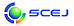## \$B9V1i%W%m%0%i%`!J2q>l!&F|DxJL!K(B

English page

### D \$B2q>l!&Bh(B 1 \$BF|(B

\$B:G=*99?7F|;~!'(B2019-12-10 12:54:57
\$B9V1i(B
\$B;~9o(B
\$B9V1i(B
\$BHV9f(B
\$B9V1iBjL\!?H/I=\$B%-!<%o!<%I(B\$BJ,N`(B
\$BHV9f(B
\$B\$BHV9f(B
\$BN3;R!&N.BN%W%m%;%9(B
(10:00\$B!A(B11:40) (\$B:BD9(B \$B0KF#(B \$BOB9(!&;0Ln(B \$BBY;V(B)
10:00\$B!A(B 10:20D104\$B%=%U%H%&%'%"(BOpenFOAM\$B\$r;HMQ\$7\$?G4CF@-1UBN\$N<+M33&LLN.\$l2r@O(B
(\$BJ<8K8)Bg(B) (\$B3X(B)\$B!{?"B<(B \$BAoIp(B\$B!&(B (\$B@5(B)\$B0KF#(B \$BOB9((B\$B!&(B (\$B@5(B)\$BED8}(B \$BfF8g(B\$B!&(B (\$B@5(B)\$B;3K\(B \$BBs;J(B\$B!&(B (\$B@5(B)\$BA0ED(B \$B8w<#(B
viscoelastic fluid
Coating flow
OpenFOAM
2-a16
10:20\$B!A(B 10:40D105\$B>.7?%(%"%j%U%H%]%s%W\$N4pAC2A%b%G%k(B
(\$BJ<8K8)Bg(B) (\$B3X(B)\$B!{>e7n(B \$BD>?M(B\$B!&(B (\$B@5(B)\$B0KF#(B \$BOB9((B\$B!&(B (\$B%"%\$%(%s%9(B) \$B5HED(B \$B7{;K(B\$B!&(B (\$BJ<8K8)Bg(B) (\$B@5(B)\$BED8}(B \$BfF8g(B\$B!&(B (\$B@5(B)\$B;3K\(B \$BBs;J(B\$B!&(B (\$B@5(B)\$BA0ED(B \$B8w<#(B
airlift pump
Two-pahse flow
Aeration pipe
2-a17
10:40\$B!A(B 11:00D106\$B?eCf>.7?%(%"%l!<%?\$K\$h\$kHy:YAtN`\$NG]M\(B
(\$BJ<8K8)Bg(B) (\$B3X(B)\$B!{2,K\(B \$BBYCN(B\$B!&(B (\$B@5(B)\$B0KF#(B \$BOB9((B\$B!&(B (\$B%"%\$%(%s%9(B) \$B5HED(B \$B7{;K(B\$B!&(B (\$BJ<8K8)Bg(B) (\$B@5(B)\$BED8}(B \$BfF8g(B\$B!&(B (\$B@5(B)\$B;3K\(B \$BBs;J(B\$B!&(B (\$B@5(B)\$BA0ED(B \$B8w<#(B
microalgae
open pond cultivation
underwater aerator
2-a18
11:00\$B!A(B 11:20D107\$BJ4BN05=L@.7A;~\$N6b7?FbDlLL\$*\$h\$SB&LL\$X\$NEAGE05NO\$K5Z\$\\$9J4BNFC@-\$N1F6A(B
(\$B2,;3Bg1!<+(B) (\$B3X(B)\$B!{>.NS(B \$BN\$B!&(B (\$B@5(B)\$B8eF#(B \$BK.>4(B\$B!&(B (\$B@5(B)\$B;0Ln(B \$BBY;V(B\$B!&(B (\$B@5(B)\$BCfA>(B \$B9@0l(B
compression
powder
2-f109
11:20\$B!A(B 11:40D108\$BJ4BNYxYB%W%m%;%9\$K\$*\$1\$kE:2C1UBN\$NJ,;65sF0\$K5Z\$\\$9G4EY\$N1F6A(B
(\$B2,;3Bg1!<+(B) (\$B3X(B)\$B!{?7AR(B \$B4]Li(B\$B!&(B (\$B@5(B)\$B;0Ln(B \$BBY;V(B\$B!&(B (\$B@5(B)\$BCfA>(B \$B9@0l(B\$B!&(B (\$B@5(B)\$B8eF#(B \$BK.>4(B
Powder(C) 2019 \$B8x1W
E-mail: inquiry-ks2019www3.scej.org
This page was generated byeasp 2.44; proghtml 2.42 (C)1999-2018 kawase